### Practice Set 7.3 Mensuration Class 10th Mathematics Part 2 MHB Solution

Practice Set 7.3

1. Radius of a circle is 10 cm. Measure of an arc of the circle is 54°. Find the area of…
2. Measure of an arc of a circle is 80 cm and its radius is 18 cm. Find the length of the…
3. Radius of a sector of a circle is 3.5 cm and length of its arc is 2.2 cm. Find the area…
4. Radius of a circle is 10 cm. Area of a sector of the sector is 100 cm^2 . Find the area…
5. Area of a sector of a circle of radius 15 cm is 30 cm^2 . Find the length of the arc of…
6. In the figure 7.31, radius of the circle is 7 cm and m(arc MBN) = 60°,find (1) Area of…
7. In figure 7.32, radius of circle is 3.4 cm and perimeter of sector P-ABC is 12.8 cm.…
8. In figure 7.33 O is the centre of the sector.∠ ROQ = ∠ MON = 60°. OR = 7 cm, and OM =…
9. In figure 7.34, if A(P-ABC) = 154 cm^2 radius of the circle is 14cm, find (1) ∠ APC.…
10. Radius of a sector of a circle is 7 cm. If measure of arc of the sector is - (l) 30°…
11. The area of a minor sector of a circle is 3.85 cm^2 and the measure of its central…
12. In figure 7.35, □PQRS is a rectangle. If PQ = 14 cm, QR = 21 cm, find the areas of the…
13. ∆ LMN is an equilateral triangle. LM = 14 cm. As shown in figure, three sectors are…

###### Practice Set 7.3
Question 1.

Radius of a circle is 10 cm. Measure of an arc of the circle is 54°. Find the area of the sector associated with the arc. (π = 3.14)

Radius of circle, r = 10 cm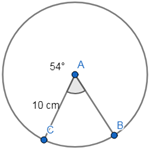Angle made between the arc ,θ = 54°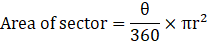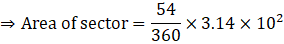⇒ Area of sector = 47.1 sq. cm

Question 2.

Measure of an arc of a circle is 80 cm and its radius is 18 cm. Find the length of the arc. (π = 3.14)

Measure of an arc of circle, θ = 80°

Radius of circle, r = 18 cm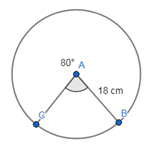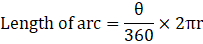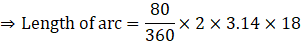⇒ Area of sector = 25.12 cm

Question 3.

Radius of a sector of a circle is 3.5 cm and length of its arc is 2.2 cm. Find the area of the sector.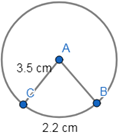Radius of circle, r = 3.5 cm

Length of arc, l = 2.2 cm

As we know,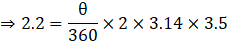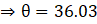Also,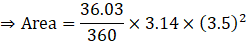⇒ Area of sector = 3.85 sq. cm

Question 4.

Radius of a circle is 10 cm. Area of a sector of the sector is 100 cm2. Find the area of its corresponding major sector. (π = 3.14)

Radius of circle, r = 10 cm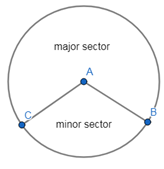Area of sector (minor sector) = 100 sq. cm

Area of circle, AC = πr2

⇒ AC = 3.14× 102

⇒ AC = 314 sq. cm

Area of major sector, AM = area of circle – area of minor sector

⇒ AM= AC – 100

⇒ AM = 314 -100 = 214 sq. cm

∴ area of major sector is 214 sq. cm

Question 5.

Area of a sector of a circle of radius 15 cm is 30 cm2. Find the length of the arc of the sector.

Radius of circle, r = 15 cm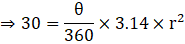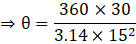Also,On substituting the values, we get,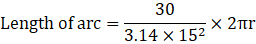⇒ Length of arc = 4 cm

Question 6.

In the figure 7.31, radius of the circle is 7 cm and m(arc MBN) = 60°,find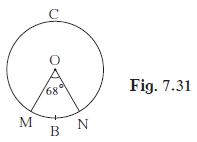(1) Area of circle

(2) A(O – MBN)

(3) A(O - MCN)

(1) Radius of circle, r = 7cm

Area of circle,AC = πr2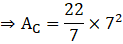⇒ AC = 154 sq. cm

∴ area of circle is 154 sq.cm

(2) Angle subtended by the arc = 60°

As we know,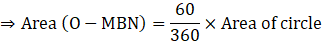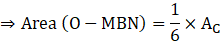⇒ A(O- MBN) = 25.7 sq. cm

(3) A(O- MCN) = Area of circle – A(O – MBN)

⇒ Area(O – MCN) = AC – 25.7

⇒ Area(O – MCN) = 154 – 25.7

∴ Area of major sector is 128.3 sq. cm

Question 7.

In figure 7.32, radius of circle is 3.4 cm and perimeter of sector P-ABC is 12.8 cm. Find A (P-ABC).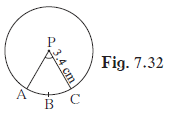Radius of circle, r = 3.4 cm

Perimeter of sector, P = 12.8

⇒ P = length of arc + 2× radius

⇒ Length of arc, l = P – 2×r

⇒ l = 12.8 – 2(3.4)

⇒ l = 6 cm

Let the ∠ APC be θ

As we know that,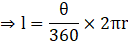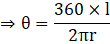Also ,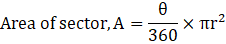On Substituting the value of theta from above equation,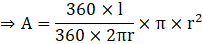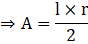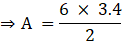⇒ A = 10.2 sq. cm

∴ Area of Sector is 10.2 sq. cm

Question 8.

In figure 7.33 O is the centre of the sector.∠ ROQ = ∠ MON = 60°. OR = 7 cm, and OM = 21 cm. Find the lengths of arc RXQ and arc MYN.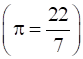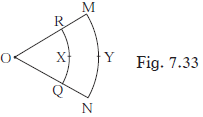Let the ∠ ROQ= ∠ MON = θ = 60°

As we know that,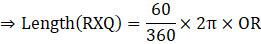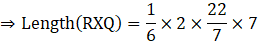⇒ Length(RXQ) = 7.6 cm

Similarly,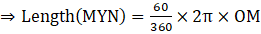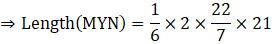⇒ Length (MYN) = 22 cm

Question 9.

In figure 7.34, if A(P-ABC) = 154 cm2radius of the circle is 14cm, find

(1) ∠ APC.

(2) l (arc ABC).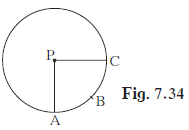As we know that,(1) Let the ∠APC be θ

Radius of circle, r =14 cm

Area of sector, A = 154 cm2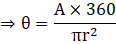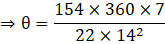⇒ θ = 90°

(2) Since the angle formed is 90° , which is one–fourth of the perimeter of circle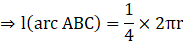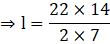⇒ l = 22 cm

Question 10.

Radius of a sector of a circle is 7 cm. If measure of arc of the sector is –

(l) 30°

(ll) 210°

(lll) three right angles

Find the area of sector in each case.

Radius of circle, r = 7cm

(l) Angle subtended by arc, θ = 30°

As we know,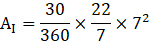⇒ Al = 12. 83 sq. cm

(ll) Angle subtended by arc, θ = 210°

Similarly,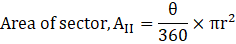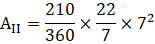⇒ All = 89.83 sq. cm

(lll) Angle subtended by arc, θ = (3× 90)° = 270°

Similarly,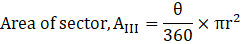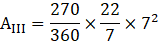⇒ Alll = 115.5 sq. cm

Question 11.

The area of a minor sector of a circle is 3.85 cm2 and the measure of its central angle is 36°. Find the radius of the circle.

As we know that,Given area of sector, A = 3.85 sq.cm

Central angle, θ = 36°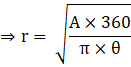On substituting the values, we get,

⇒ r = 3.5 cm

∴ Radius of circle is 3.5 cm

Question 12.

In figure 7.35, □PQRS is a rectangle. If PQ = 14 cm, QR = 21 cm, find the areas of the parts x, y and z.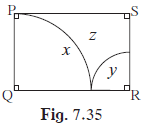Since part x is a sector of a circle with radius, r = 14 cm and the central angle is 90°, so the area of x will be equal to one-fourth of the area of circle with PQ as radius.

Area of circle with PQ as radius = π (PQ)2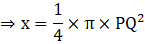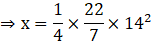⇒ x = 154 sq. cm

Similarly, area y is also equal to one-fourth od area of circle with radius, r = QR – PQ

⇒ r = 21 – 14 = 7 cm

Area of circle with r as radius = π (r)2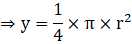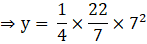⇒ y = 38.5 sq. cm

Also,

z = Area of rectangle(PQRS) – x – y

Area of rectangle = PQ× QR

⇒ Area of rectangle = 14× 21 = 294 sq. cm

⇒ z = 294 – 154 – 38.5

⇒ z = 101.5 sq.cm

∴ the area of x, y and z are 154 sq.cm, 38.5 sq.cm and 101.5 sq.cm respectively

Question 13.

∆ LMN is an equilateral triangle. LM = 14 cm. As shown in figure, three sectors are drawn with vertices as centre and radius7 cm. Find,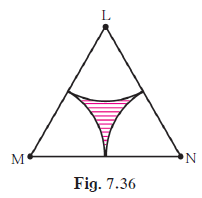(1) A (Δ LMN)

(2) Area of any one of the sectors

(3) Total area of all three sectors

(1) Side of triangle = LM = a = 14 cm

Since Δ LMN is an equilateral triangle, so the area of the triangle is given by: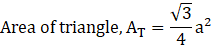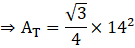⇒ AT = 84.87 sq.cm

(2) Angle subtended by the corner = θ = 60°

As we know,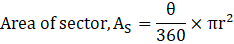Here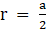,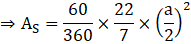⇒ AS = 25.67 sq. cm

(3) Total area of all sector, ATS = 3× AS

⇒ ATS = 3× 25.67

⇒ ATS = 77.01 sq.cm

(4) Area of shaded region, AR = Area of triangle – Area of all three sectors

⇒ AS = AT - ATS

⇒ AS = 84.87 – 77.01

⇒ AS = 7.86 sq. cm

∴ area of shaded region is 7.86 sq. cm

## PDF FILE TO YOUR EMAIL IMMEDIATELY PURCHASE NOTES & PAPER SOLUTION. @ Rs. 50/- each (GST extra)

SUBJECTS

HINDI ENTIRE PAPER SOLUTION

MARATHI PAPER SOLUTION
SSC MATHS I PAPER SOLUTION
SSC MATHS II PAPER SOLUTION
SSC SCIENCE I PAPER SOLUTION
SSC SCIENCE II PAPER SOLUTION
SSC ENGLISH PAPER SOLUTION
SSC & HSC ENGLISH WRITING SKILL
HSC ACCOUNTS NOTES
HSC OCM NOTES
HSC ECONOMICS NOTES
HSC SECRETARIAL PRACTICE NOTES

2019 Board Paper Solution

HSC ENGLISH SET A 2019 21st February, 2019

HSC ENGLISH SET B 2019 21st February, 2019

HSC ENGLISH SET C 2019 21st February, 2019

HSC ENGLISH SET D 2019 21st February, 2019

SECRETARIAL PRACTICE (S.P) 2019 25th February, 2019

HSC XII PHYSICS 2019 25th February, 2019

CHEMISTRY XII HSC SOLUTION 27th, February, 2019

OCM PAPER SOLUTION 2019 27th, February, 2019

HSC MATHS PAPER SOLUTION COMMERCE, 2nd March, 2019

HSC MATHS PAPER SOLUTION SCIENCE 2nd, March, 2019

SSC ENGLISH STD 10 5TH MARCH, 2019.

HSC XII ACCOUNTS 2019 6th March, 2019

HSC XII BIOLOGY 2019 6TH March, 2019

HSC XII ECONOMICS 9Th March 2019

SSC Maths I March 2019 Solution 10th Standard11th, March, 2019

SSC MATHS II MARCH 2019 SOLUTION 10TH STD.13th March, 2019

SSC SCIENCE I MARCH 2019 SOLUTION 10TH STD. 15th March, 2019.

SSC SCIENCE II MARCH 2019 SOLUTION 10TH STD. 18th March, 2019.

SSC SOCIAL SCIENCE I MARCH 2019 SOLUTION20th March, 2019

SSC SOCIAL SCIENCE II MARCH 2019 SOLUTION, 22nd March, 2019

XII CBSE - BOARD - MARCH - 2019 ENGLISH - QP + SOLUTIONS, 2nd March, 2019

HSC Maharashtra Board Papers 2020

(Std 12th English Medium)

HSC ECONOMICS MARCH 2020

HSC OCM MARCH 2020

HSC ACCOUNTS MARCH 2020

HSC S.P. MARCH 2020

HSC ENGLISH MARCH 2020

HSC HINDI MARCH 2020

HSC MARATHI MARCH 2020

HSC MATHS MARCH 2020

SSC Maharashtra Board Papers 2020

(Std 10th English Medium)

English MARCH 2020

HindI MARCH 2020

Hindi (Composite) MARCH 2020

Marathi MARCH 2020

Mathematics (Paper 1) MARCH 2020

Mathematics (Paper 2) MARCH 2020

Sanskrit MARCH 2020

Important-formula

THANKS